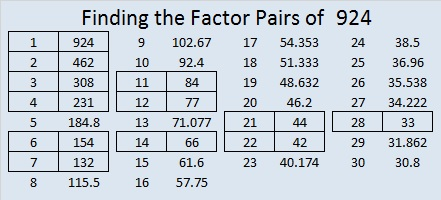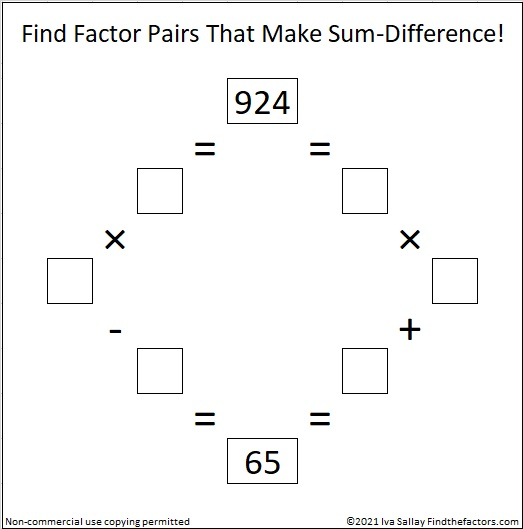# Applying Divisibility Rules to 924

Contents

### Divisibility Rules and 924:

Let’s apply some basic divisibility rules to find some of the factors of 924:

1. Like every other counting number, 924 is divisible by 1.
2. Since 924 is even, it is divisible by 2.
3. 9 + 2 + 4 = 15, a number divisible by 3, so 924 is divisible by 3.
4. Its last two digits, 24, is divisible by 4, so 924 is divisible by 4.
5. Its last digit isn’t 0 or 5, so 924 is NOT divisible by 5.
6. 924 is even and divisible by 3, so it is also divisible by 6.
7. Since 92-2(4) = 84, a number divisible by 7, we know that 924 is also divisible by 7.
8. Since its last two digits are divisible by 8, and the third to the last digit, 9, is odd, 924 is NOT divisible 8.
9. 9 + 2 + 4 = 15, a number not divisible by 9, so 924 is NOT divisible by 9.
10. The last digit is not 0, so 924 is NOT divisible by 10.
11. 9 – 2 + 4 = 11, so 924 is divisible by 11.

Thus, 1, 2, 3, 4, 6, 7, and 11 are all factors of 924.

### Factor Cake for 924:

You can see its prime factors easily on the outside of its festive prime factor cake:### Factors of 924:

• 924 is a composite number.
• Prime factorization: 924 = 2 x 2 x 3 x 7 x 11, which can be written 924 = 2² x 3 x 7 x 11
• The exponents in the prime factorization are 2, 1, 1, and 1. Adding one to each and multiplying we get (2 + 1)(1 + 1)(1 + 1)(1 + 1) = 3 x 2 x 2 x 2 = 24. Therefore 924 has exactly 24 factors.
• Factors of 924: 1, 2, 3, 4, 6, 7, 11, 12, 14, 21, 22, 28, 33, 42, 44, 66, 77, 84, 132, 154, 231, 308, 462, 924
• Factor pairs: 924 = 1 x 924, 2 x 462, 3 x 308, 4 x 231, 6 x 154, 7 x 132, 11 x 84, 12 x 77, 14 x 66, 21 x 44, 22 x 42, or 28 x 33,
• Taking the factor pair with the largest square number factor, we get √924 = (√4)(√231) = 2√231 ≈ 30.3973683.### Sum Difference Puzzle:

924 has twelve factor pairs. One of the factor pairs adds up to 65, and a different one subtracts to 65. If you can identify those factor pairs, then you can solve this puzzle!### More about the Number 924:

You may have seen one of its many possible factor trees contained in the first frame of this factor tree for 852,852 from my previous post:924 is in the very center of the 12th row of Pascal’s triangle because 12!/(6!6!) = 924.924 is the sum of consecutive prime numbers: 461 + 463 = 924

I like 924 written in some other bases:
770 BASE 11
336 BASE 17
220 BASE 21
SS BASE 32, S is 28
S0 BASE 33

924 has several sets of consecutive factors. Besides being divisible by the 1st, 2nd, and 3rd triangular numbers (1, 3, and 6), those consecutive factors mean the following:

• 924 is divisible by the 6th triangular number, 21, which is 6(7)/2.
• 924 is divisible by the 11th triangular number, 66, which is 11(12)/2.
• 924 is divisible by the 21st triangular number, 231, which is 21(22)/2.

This site uses Akismet to reduce spam. Learn how your comment data is processed.